# Stock market trends and Value at Risk¶This notebook is an element of the risk-engineering.org courseware. It can be distributed under the terms of the Creative Commons Attribution-ShareAlike licence.

Author: Eric Marsden [email protected]

This notebook contains an introduction to the use of SciPy library’s support for fitting probability distributions, and the Panda library’s support for manipulating stock market data. See the accompanying lecture slides for background on the use of Value at Risk (VaR) as a financial risk metric.

Warning: the analysis given here is pedagogical in nature and is not suitable for investment purposes!

In :
import numpy
import scipy.stats
import matplotlib.pyplot as plt
import pandas
from datetime import datetime
%config InlineBackend.figure_formats=['png']


## Stock market returns and volatility¶

It's a little fiddly to obtain free access to historical data on stock market prices. For US stocks, you can use Quandl if you register on their web site to obtain an API token (it's free). At the beginning of 2019, it's possible to download data from Yahoo Finance, thanks to the yahoofinancials library which you will need to install with a command such as

sudo pip3 install yahoofinancials

Here is a function that obtains stock data and places it in a pandas DataFrame so that we can analyze it.

In :
from yahoofinancials import YahooFinancials

def retrieve_stock_data(ticker, start, end):
json = YahooFinancials(ticker).get_historical_price_data(start, end, 'daily')
df = pandas.DataFrame(columns=['open','close','adjclose'])
for row in json[ticker]['prices']:
date = datetime.fromisoformat(row['formatted_date'])
df.loc[date] = [row['open'], row['close'], row['adjclose']]
df.index.name = "date"
return df


Let’s download some stock market data for Microsoft (stock market ticker is MSFT) for the year 2018 and plot its evolution over time. We plot the adjusted close, which means the price at the close of each day of trading, adjusted for any events such as dividends, stock splits and new stock offerings during that day.

In :
MSFT = retrieve_stock_data("MSFT", "2018-01-01", "2019-01-01")
MSFT.plot(y="adjclose")
plt.title("Microsoft stock in 2018", weight='bold');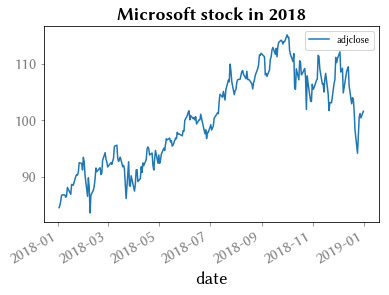For risk analysis we are more interested in daily changes than in the absolute value of the stock, so we plot the daily returns on the stock.

In :
fig = plt.figure()
fig.set_size_inches(10,3)
MSFT["adjclose"].pct_change().plot()
plt.title("MSFT daily returns in 2018", weight='bold');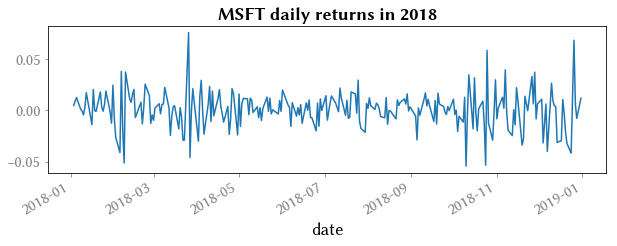In :
MSFT["adjclose"].pct_change().hist(bins=50, density=True, histtype='stepfilled', alpha=0.5)
plt.title("Histogram of MSFT daily returns in 2018", weight='bold')
MSFT["adjclose"].pct_change().std()

Out:
0.01783865195923279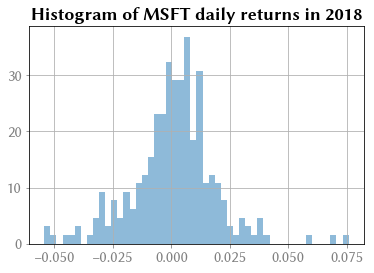Let’s see whether the daily returns are better represented by a normal distribution or by a Student-t distribution.

In :
Q = MSFT["adjclose"].pct_change().dropna()
scipy.stats.probplot(Q, dist=scipy.stats.norm, plot=plt.figure().add_subplot(111))
plt.title("Normal QQ-plot of MSFT daily returns in 2018", weight="bold");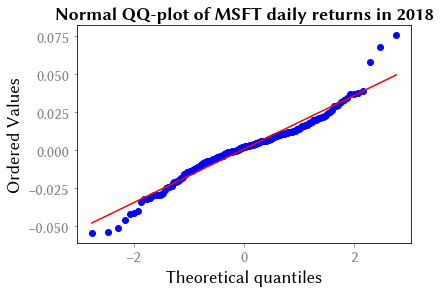In :
tdf, tmean, tsigma = scipy.stats.t.fit(Q)
scipy.stats.probplot(Q, dist=scipy.stats.t, sparams=(tdf, tmean, tsigma), plot=plt.figure().add_subplot(111))
plt.title("Student QQ-plot of MSFT daily returns in 2018", weight="bold");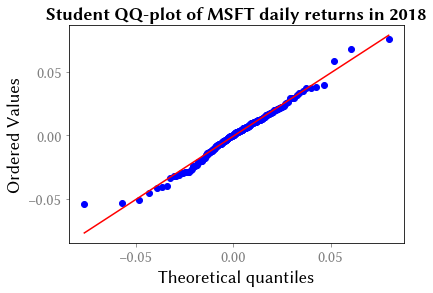Student’s t distribution does seem to fit better (look in particular at the tails of the distribution).

## Value at Risk using the historical bootstrap method¶

Method: we calculate empirical quantiles from a histogram of daily returns. Let’s do that for the returns of Halliburton (stock market ticker HAL) over 2010–2014 (in reality, you would look at a longer time horizon, and give more weight to recent activity than to activity several years ago).

In :
stock = retrieve_stock_data("HAL", "2010-01-01", "2014-01-01")
returns = stock["adjclose"].pct_change().dropna()
mean = returns.mean()
sigma = returns.std()
tdf, tmean, tsigma = scipy.stats.t.fit(returns)
returns.hist(bins=40, density=True, histtype='stepfilled', alpha=0.5)
plt.title("Daily returns on HAL, 2010-2014", weight="bold");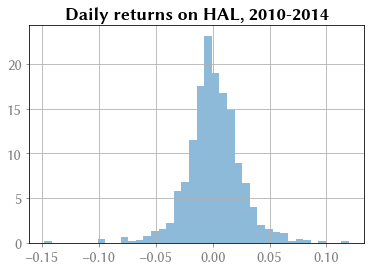In :
returns.quantile(0.05)

Out:
-0.034135096442180116

The 0.05 empirical quantile of daily returns is at -0.034. That means that with 95% confidence, our worst daily loss will not exceed 3.4%. If we have a 1 M€ investment, our one-day 5% VaR is 0.034 * 1 M€ = 34 k€.

Exercise: estimate the one-day 1% VaR for the same stock using the historical bootstrap method.

## Value at Risk using the variance-covariance method¶

Method: calculate analytic quantiles by curve fitting to historical data. Here, we use Student’s t distribution (we checked above that it represents daily returns relatively well).

In :
support = numpy.linspace(returns.min(), returns.max(), 100)
returns.hist(bins=40, density=True, histtype='stepfilled', alpha=0.5);
plt.plot(support, scipy.stats.t.pdf(support, loc=tmean, scale=tsigma, df=tdf), "r-")
plt.title("Daily change in HAL over 2010–2014 (%)", weight='bold');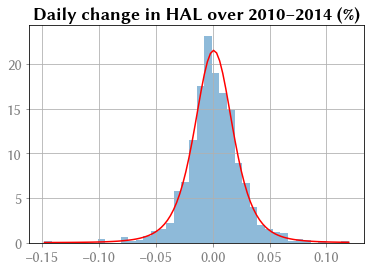In :
scipy.stats.norm.ppf(0.05, mean, sigma)

Out:
-0.038358372194607256

Our analytic 0.05 quantile is at -0.0384, so with 95% confidence, our worst daily loss will not exceed 3.84%. For a 1 M€ investment, one-day Value at Risk is 0.0384 * 1 M€ = 38 k€.

Exercise: estimate the one-day Value at Risk at 1% confidence level for 1 M€ invested in Apple stock (ticker is AAPL).

## Value at Risk using the Monte Carlo method¶

Method: run many trials with random market conditions, calculating portfolio loss for each trial. Use the aggregated trial data to establish a profile of the portfolio’s risk characteristics. See the slides associated with this notebook for details.

Hypothesis: stock market evolution can be simulated by geometric Brownian motion (this is a rough hypothesis; see the literature on stochastic methods in finance for more sophisticated, and more realistic, models, such as jump diffusion).

We start by defining some parameters of the geometric Brownian motion.

In :
days = 300   # time horizon
dt = 1/float(days)
sigma = 0.04 # volatility
mu = 0.05  # drift (average growth rate)


This function simulates one stock market evolution, and returns the price evolution as an array. It simulates geometric Brownian motion using pseudorandom numbers drawn from a normal distribution (see accompanying slides).

In :
def random_walk(startprice):
price = numpy.zeros(days)
shock = numpy.zeros(days)
price = startprice
for i in range(1, days):
shock[i] = numpy.random.normal(loc=mu * dt, scale=sigma * numpy.sqrt(dt))
price[i] = max(0, price[i-1] + shock[i] * price[i-1])
return price


Let’s simulate 30 random walks, starting from an initial stock price of 10€, for a duration of 300 days.

In :
for run in range(30):
plt.plot(random_walk(10.0))
plt.xlabel("Time")
plt.ylabel("Price");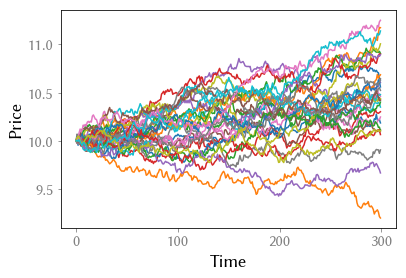Final price is spread out between 9.5€ (our portfolio has lost value) to almost 12€. The expectation (mean outcome) is a profit; this is due to the fact that the drift in our random walk (parameter mu) is positive.

Now let’s run a big Monte Carlo simulation of random walks of this type, to obtain the probability distribution of the final price, and obtain quantile measures for the Value at Risk estimation. This will take a little time to run (decrease variable runs if you want faster, but less representative, results).

In :
runs = 10000
simulations = numpy.zeros(runs)
for run in range(runs):
simulations[run] = random_walk(10.0)[days-1]
q = numpy.percentile(simulations, 1)
plt.hist(simulations, density=True, bins=30, histtype='stepfilled', alpha=0.5)
plt.figtext(0.6, 0.8, "Start price: 10€")
plt.figtext(0.6, 0.7, "Mean final price: {:.3}€".format(simulations.mean()))
plt.figtext(0.6, 0.6, "VaR(0.99): {:.3}€".format(10 - q))
plt.figtext(0.15, 0.6, "q(0.99): {:.3}€".format(q))
plt.axvline(x=q, linewidth=4, color='r')
plt.title("Final price distribution after {} days".format(days), weight='bold');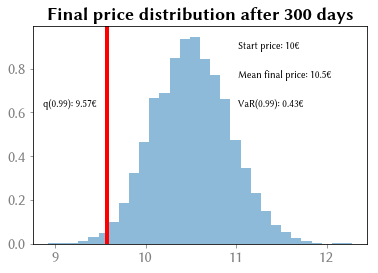We have looked at the 1% empirical quantile of the final price distribution to estimate the Value at Risk, which is 0.427€ for a 10€ investment.

## Correlation between stocks¶

Let's examine the variation of stock indexes in different countries: the CAC40 index which summarizes the price of the 40 largest listed companies on the Paris stock exchange, the DAX which does likewise for the German stock exchange, the Hang Send Index in Hong Kong and finally the All Ordinaries in Australia.

We are interested in checking whether these stock indexes are correlated: when one goes down, does the other also tend to move in the same direction? See our course notes for the importance of correlation in financial risk analysis.

In :
start = "2005-01-01"
end   = "2010-01-01"
CAC = retrieve_stock_data("^FCHI", start, end)
DAX = retrieve_stock_data("^GDAXI", start, end)
HSI = retrieve_stock_data("^HSI", start, end)
AORD = retrieve_stock_data("^AORD", start, end)

df = pandas.DataFrame({ 'CAC': CAC["adjclose"].pct_change(),
'DAX': DAX["adjclose"].pct_change(),
'HSI': HSI["adjclose"].pct_change(),
'AORD': AORD["adjclose"].pct_change() })
dfna = df.dropna()

In :
plt.plot(dfna["CAC"], dfna["DAX"], 'r.', alpha=0.5)
plt.xlabel("CAC40 daily return")
plt.ylabel("DAX daily return")
plt.title("CAC vs DAX daily returns, 2005–2010", weight='bold');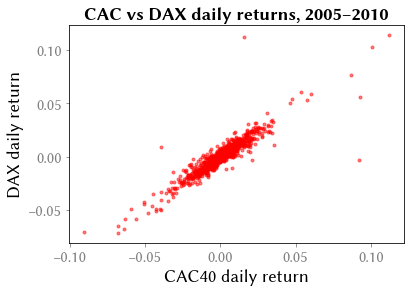In :
scipy.stats.pearsonr(dfna["CAC"], dfna["DAX"])

Out:
(0.9176923741808769, 0.0)

This is a high level of correlation between the French and German indexes. We can interpret this as indicating that large French and German firms are exposed to quite similar opportunities and threats.

In :
plt.plot(dfna["CAC"], dfna["AORD"], 'r.', alpha=0.5)
plt.xlabel("CAC40 daily return")
plt.ylabel("All Ordinaries daily return")
# ensure square aspect ratio
plt.axes().set_xlim(-0.15, 0.15)
plt.axes().set_ylim(-0.15, 0.15)
plt.title("CAC vs All Ordinaries index daily returns, 2005–2010", weight='bold');

/usr/lib/python3/dist-packages/matplotlib/figure.py:98: MatplotlibDeprecationWarning:
Adding an axes using the same arguments as a previous axes currently reuses the earlier instance.  In a future version, a new instance will always be created and returned.  Meanwhile, this warning can be suppressed, and the future behavior ensured, by passing a unique label to each axes instance.
"Adding an axes using the same arguments as a previous axes "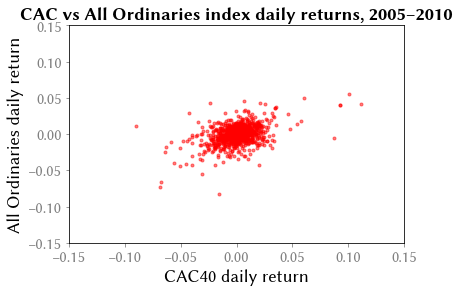In :
scipy.stats.pearsonr(dfna["CAC"], dfna["AORD"])

Out:
(0.4293677191097516, 7.833870901667016e-51)

Here the level of correlation is much lower: the influences on large French and Australian firms are fairly different.

In :
returns = dfna["CAC"]
returns.hist(bins=30, density=True, histtype='stepfilled', alpha=0.5)
support = numpy.linspace(returns.min(), returns.max(), 100)
tdf, tmean, tsigma = scipy.stats.t.fit(returns)
print("CAC t fit: mean={}, scale={}, df={}".format(tmean, tsigma, tdf))
plt.plot(support, scipy.stats.t.pdf(support, loc=tmean, scale=tsigma, df=tdf), "r-")
plt.figtext(0.6, 0.7, "tμ = {:.3}".format(tmean))
plt.figtext(0.6, 0.65, "tσ = {:.3}".format(tsigma))
plt.figtext(0.6, 0.6, "df = {:.3}".format(tdf))
plt.title("Histogram of CAC40 daily returns over 2005–2010", weight='bold');

CAC t fit: mean=0.0005614840639463816, scale=0.008993220376494136, df=2.7622265665053067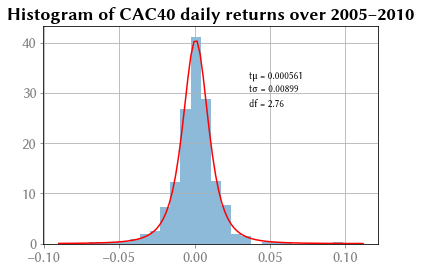In :
returns = dfna["DAX"]
returns.hist(bins=30, density=True, histtype='stepfilled', alpha=0.5)
support = numpy.linspace(returns.min(), returns.max(), 100)
tdf, tmean, tsigma = scipy.stats.t.fit(returns)
print("DAX t fit: mean={}, scale={}, df={}".format(tmean, tsigma, tdf))
plt.plot(support, scipy.stats.t.pdf(support, loc=tmean, scale=tsigma, df=tdf), "r-")
plt.figtext(0.6, 0.7, "tμ = {:.3}".format(tmean))
plt.figtext(0.6, 0.65, "tσ = {:.3}".format(tsigma))
plt.figtext(0.6, 0.6, "df = {:.3}".format(tdf))
plt.title("Histogram of DAX daily returns over 2005–2010", weight='bold');

DAX t fit: mean=0.0009649852388805224, scale=0.008832752965674647, df=2.7514125614261165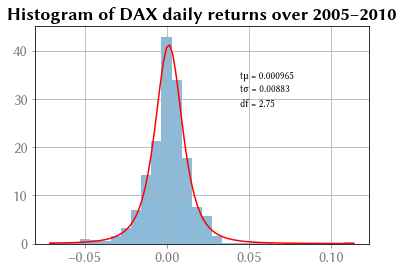Let’s simulate random joint stock returns from a joint distribution of fitted-CAC and fitted-DAX according to the distribution parameters estimated above, and plot the results. We need these simulated joint returns to do a Monte Carlo VaR analysis of a stock portfolio composed of CAC and DAX stock.

In :
runs = 5000
fittedCAC = numpy.zeros(runs, float)
fittedDAX = numpy.zeros(runs, float)
for i in range(runs):
fittedCAC[i] = scipy.stats.t.rvs(loc=0.000478137351981, scale=0.00898201242824, df=2.75557323986)
fittedDAX[i] = scipy.stats.t.rvs(loc=0.000847802944791, scale=0.00878082895409, df=2.71766905436)
plt.plot(fittedCAC, fittedDAX, 'r.', alpha=0.5)
plt.title("CAC vs DAX returns (simulated, no correlation)", weight='bold');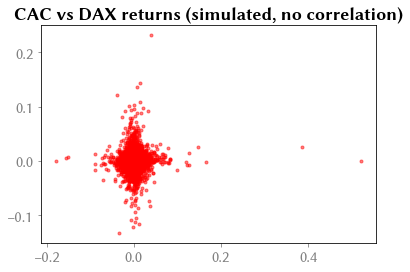Problem: our sampling from these fitted random variables doesn’t match the observations from the real markets (scroll up to check the real CAC/DAX scatterplot).

We need some way of generating a sample that respects the correlation between the input variables. All is revealed in the next episode on Copula and multivariate dependencies.